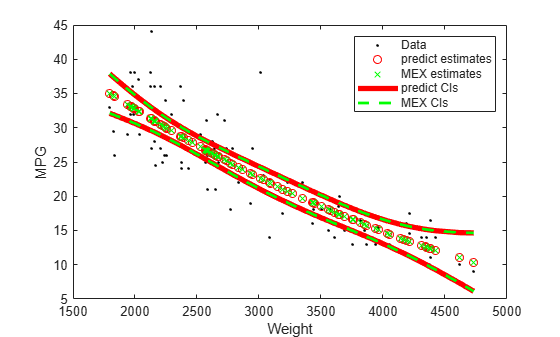Documentación

Esta página aún no se ha traducido para esta versión. Puede ver la versión más reciente de esta página en inglés.

# predict

Clase: CompactLinearModel

Predict response of linear regression model

## Sintaxis

```ypred = predict(mdl,Xnew) [ypred,yci] = predict(mdl,Xnew) [ypred,yci] = predict(mdl,Xnew,Name,Value) ```

## Description

`ypred = predict(mdl,Xnew)` returns the predicted response of the `mdl` linear regression model to the points in `Xnew`.

```[ypred,yci] = predict(mdl,Xnew)``` returns confidence intervals for the true mean responses.

```[ypred,yci] = predict(mdl,Xnew,Name,Value)``` predicts responses with additional options specified by one or more `Name,Value` pair arguments.

expandir todo

Linear model object, specified as a full `LinearModel` object constructed using `fitlm` or `stepwiselm`, or a compacted `CompactLinearModel` object constructed using `compact`.

New predictor input values, specified as a table, dataset array, or numeric matrix.

• If `Xnew` is a table or dataset array, it must contain the predictor names in `mdl`.

• If `Xnew` is a numeric matrix, it must have the same number of variables (columns) as was used to create `mdl`. Furthermore, all variables used in creating `mdl` must be numeric.

### Argumentos de par nombre-valor

Specify optional comma-separated pairs of `Name,Value` arguments. `Name` is the argument name and `Value` is the corresponding value. `Name` must appear inside quotes. You can specify several name and value pair arguments in any order as `Name1,Value1,...,NameN,ValueN`.

Alpha value for confidence interval, specified as the comma-separated pair consisting of `'Alpha'` and a numeric value in the range [0,1]. The confidence level of `yci` is equal to 100(1–alpha)%. For example, specifying `Alpha` as 0.05 corresponds to a 95% confidence interval.

Prediction type, specified as the comma-separated pair consisting of `'Prediction'` and one of the following.

• `'curve'``predict` predicts confidence bounds for the fitted mean values.

• `'observation'``predict` predicts confidence bounds for the new observations. This results in wider bounds because the error in a new observation is equal to the error in the estimated mean value, plus the variability in the observation from the true mean.

For details, see `polyconf`.

Flag to compute simultaneous confidence bounds, specified as the comma-separated pair consisting of Simultaneous and either false or true. If you specify `true`, then the function computes confidence bounds are for all predictor values simultaneously. If you specify `false`, then the function calculates confidence bounds using a hold for each individual predictor value. Simultaneous bounds are wider than separate bounds, because it is more stringent to require that the entire curve be within the bounds than to require that the curve at a single predictor value be within the bounds.

For details, see `polyconf`.

## Output Arguments

expandir todo

Predicted mean response values evaluated at `Xnew`, returned as a numeric vector. `ypred` is the same size as `Xnew`.

Confidence intervals for predicted responses, returned as a two-column matrix with each row providing one interval. The meaning of the confidence interval depends on the settings of the name-value pairs.

## Ejemplos

expandir todo

Create a model of car mileage as a function of weight, and predict the response.

Create a quadratic model of car mileage as a function of weight from the `carsmall` data.

```load carsmall X = Weight; y = MPG; mdl = fitlm(X,y,'quadratic');```

Create predicted responses to the data.

```Xnew = X; ypred = predict(mdl,Xnew);```

Plot the original responses and the predicted responses to see how they differ.

```plot(X,y,'o',Xnew,ypred,'x') legend('Data','Predictions')```This example is based on Predict Response Values Using Linear Model.

Load the `carsmall` data set, and then fit the quadratic regression model.

```load carsmall X = Weight; y = MPG; mdl = fitlm(X,y,'quadratic'); ```

Create points for prediction from the sorted in-sample data.

```Xnew = sort(X); ```

Save the fitted quadratic model to the file `QLMMdl.mat`.

```saveCompactModel(mdl,'QLMMdl'); ```

Define a function in your current working folder called `mypredictQLM.m` that:

• Accepts measurements commensurate with `Xnew` and perhaps valid name-value pair arguments

• Loads the fitted quadratic model in `QLMMdl.mat`

• Returns predictions and confidence interval bounds

```function [yhat,ci] = mypredictQLM(x,varargin) %#codegen %MYPREDICTQLM Predict response using linear model % MYPREDICTQLM predicts responses for the n observations in the n-by-1 % vector x using using the linear model stored in the MAT-file % QLMMdl.mat, and then returns the predictions in the n-by-1 vector yhat. % MYPREDICTQLM also returns confidence interval bounds for the % predictions in the n-by-2 vector ci. CompactMdl = loadCompactModel('QLMMdl'); narginchk(1,Inf); [yhat,ci] = predict(CompactMdl,x,varargin{:}); end ```

Generate a MEX function from `mypredictQLM.m`. Specify returning 90% simultaneous confidence intervals on the predictions. Because C uses static typing, `codegen` must determine the properties of all variables in MATLAB® files at compile time. To designate arguments as compile-time constants, use `coder.Constant`.

```codegen -config:mex mypredictQLM -args {Xnew,coder.Constant('Alpha'),0.1,coder.Constant('Simultaneous'),true} ```

The MEX file `mypredictQLM_mex.mexw64` generates to your present working directory. The file extension depends on your platform.

Compare predictions and confidence intervals using `predict` and `mypredictQLM_mex` by plotting both with the data. Specify name-value pair arguments in the same order as in the `-args` argument in the call to `codegen`.

```[yhat1,ci1] = predict(mdl,Xnew,'Alpha',0.1,'Simultaneous',true); [yhat2,ci2] = mypredictQLM_mex(Xnew,'Alpha',0.1,'Simultaneous',true); figure; h1 = plot(X,y,'.'); hold on; h2 = plot(Xnew,yhat1,'ro',Xnew,yhat2,'g*'); h3 = plot(Xnew,ci1,'r-','LineWidth',4); h4 = plot(Xnew,ci2,'g--','LineWidth',2); legend([h1; h2; h3(1); h4(1)],{'Data','predict estimates','MEX estimates',... 'predict CIs','MEX CIs'}); xlabel('Weight'); ylabel('MPG'); ```The generated MEX file and `predict` return essentially the same results.

## Alternatives

`feval` gives the same predictions, but uses multiple input arrays with one component in each input argument. `feval` can be simpler to use with a model created from a table or dataset array, although `feval` does not give confidence intervals on its predictions.

`random` predicts with added noise.